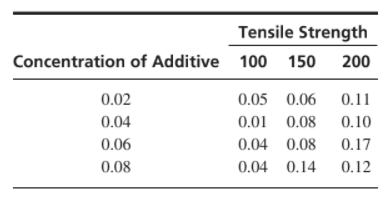×
Get Full Access to Statistics For Engineers And Scientists - 4 Edition - Chapter 2 - Problem 19se
Get Full Access to Statistics For Engineers And Scientists - 4 Edition - Chapter 2 - Problem 19se

×

# A steel manufacturer testing a new additive, forISBN: 9780073401331 38

## Solution for problem 19SE Chapter 2

Statistics for Engineers and Scientists | 4th Edition

• Textbook Solutions
• 2901 Step-by-step solutions solved by professors and subject experts
• Get 24/7 help from StudySoup virtual teaching assistantsStatistics for Engineers and Scientists | 4th Edition

4 5 1 371 Reviews
26
4
Problem 19SE

A steel manufacturer is testing a new additive for manufacturing an alloy of steel. The joint probability mass function of tensile strength (in thousands of pounds/in2) and additive concentration isa. What are the marginal probability mass functions for X (additive concentration) and Y (tensile

strength)?

b. Are X and Y independent? Explain.

c. Given that a specimen has an additive concentration of 0.04, what is the probability that its strength is 150 or more?

d. Given that a specimen has an additive concentration of 0.08, what is the probability that its tensile strength is greater than 125?

e. A certain application calls for the tensile strength to be 175 or more. What additive concentration should be used to make the probability of meeting this specification the greatest?

Step-by-Step Solution:
Step 1 of 3

Step1 of 5:

Given data states that a steel manufacturer testing a new additive, for manufacturing an alloy of steel.

The joint probability mass function of tensile strength (y) and additive concentration (x).

 Tensile strength Concentration of additive 100 150 200 0.02 0.05 0.06 0.11 0.04 0.01 0.08 0.10 0.06 0.04 0.08 0.17 0.08 0.04 0.14 0.12

Step2 of 5:

a). Here we need to find the marginal probability mass functions for X and Y.

 Tensile strength (y)Concentration of additive (x) 100 150 200 0.02 0.05 0.06 0.11 0.22 0.04 0.01 0.08 0.10 0.19 0.06 0.04 0.08 0.17 0.29 0.08 0.04 0.14 0.12 0.30.14 0.36 0.5

The marginal probability mass functionis found by summing along the rows of the joint probability mass function.

For additive concentration (x):,,,= 0 forThe marginal probability mass functionis found by summing along the columns of the joint probability mass function.

For tensile strength (y):= 0 forStep2 of 5:

b). The aim is to find, X and Y are independent or not.

In this case, X and Y are not independent.

For example,but= 0.0308.

Therefore,So, X and Y are not independent.

Step3 of 5:

c). Here we need to find the probability that its strength is 150 or more.

We have a specimen has an additive concentration of 0.04.

We use the conditional probability, that is= 0.9474

Therefore, the probability that specimen strength is 150 or more is 0.9474.

Step4 of 5:

d). To find the probability that its tensile strength is greater than 125.

We have a specimen has an additive concentration of 0.08.

We use the conditional probability, that is= 0.867

Therefore, the probability that its tensile strength is greater than 125 is 0.867.

Step5 of 5:

e). Here we need to find, what additive concentration should be used to make the probability of meeting this specification the greatest.

The tensile strength is greater than 175 if  Y

Step 2 of 3

Step 3 of 3